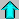# Astronomy 12 - Spring 1999 (S.T. Myers)

## Midterm 2 Review

Last update: March 24, 1999

Test Format:

This exam will concentrate on the material covered in lecture, reading, and homework since the first midterm exam. Still, you should review previous problems since the later parts of this course build upon earlier material.

As before, you will be provided with an equation sheet, and you may also bring several sheets of your own notes. I am not asking you to memorize a bunch of equations and things, so the tests (including the final) will be open-note. Because the text does things in a different, and often confusing, way, you may not use your textbook during the exam (its not open-book).

Again, the bulk of the exam (>75%) will be quantitative problems like in the homework and extra credit problem set. The remaining fraction of the exam will be qualitative. In Astro12, we will be talking about such a wide range of phenomena and measurements related to them that it will be critical to make sure we all know what we are talking about. Therefore, as part of each midterm (and the final) there will be some short answer questions where you will be asked to give a couple sentences explaining some topic (see the examples below).

The problems and questions below are for teaching and review purposes only. Do not hand in the answers for these, as you will not receive any credit!

Remember to bring a calculator.

I hope that these help to focus your studies...

Review Problems from the Textbook :

Some of the questions in the book can be used for review. The answers are not given, but you should have a pretty good idea if you know how to do a problem or not! You should at least have an idea on how to tackle problems like these.

1. Chapter 8: (p.177-178) Problems 7, 8, 9, 10, 14, 16, 17, 18.

2. Chapter 13: (p.268-269) Problems 1, 3-12, 14, 17, 18.

3. Chapter 15: (p.307-309) Problems 1, 2, 5, 9, 10, 12, 13, 14, 16, 18, 19.

4. Chapter 16: (p.330-331) Problems 1-3, 5-10, 12.

5. Chapter 17: (p.349-351) Problems 1-6, 8-13, 18-20.

This is a list of short topical questions you should be able to answer. Some of these will be on the exam. I am looking for a sentence or two, and these are qualitative, not quantitative (read as: don't memorize numbers, just know the cartoon pictures). The key topics are in italics. It is also a good idea to look through the questions posed in the Investigations section of the on-line Lecture Notes for each lecture.

1. What is the Boltzmann equation used for?

2. What is the Saha equation used for?

3. What is a Boltzmann factor and what does it signify?

4. Why does the strength of the Balmer lines observed in stellar atmospheres first increase and then decrease with decreasing temperature, reaching a maximum around 10000 K?

5. What do we mean by optical depth?

6. What does the opacity of a gas signify?

7. What is the H- ion?

8. What are the four main equations of stellar structure (do not write them down, but list them by what derivative they signify and a short description of their meanings)?

9. Why does electromagnetic radiation have pressure, even if photons have no mass?

10. What is an equation of state?

11. When do you use a convective temperature gradient instead of a radiative temperature gradient when solving the energy transport in a star?

12. Where does the main-sequence mass-luminosity relation come from?

13. What is dust extinction and reddening?

14. What is the interstellar medium?

15. What is an H II region?

16. What is the 21-cm line and what is it useful for?

17. What are molecular clouds and what are they the site of?

18. What equilibrium is assumed when computing properties of two different phases (clouds) coexisting in the interstellar medium?

19. What are the main stages of protostellar collapse?

20. Why does the fusion of hydrogen to helium give off energy?

21. Why does the fission of Uranium give off energy?

22. What do solar neutrinos signify?

23. What are the proton-proton chain, the CNO cycle, and the Triple-alpha process?

24. What is happening in a star on the red giant branch?

25. What is the helium flash?

26. What is degeneracy?

27. What is happening in a star on the asymptotic giant branch?

28. What is a planetary nebula?

29. What is a white dwarf and what are they made of?

30. What is the Chandrasekhar mass?

31. What is the heaviest element that the core of a star can be made of and why?

32. What happens when a massive > 1.4 Msun degenerate iron core forms in a massive evolved star?

33. Why was the detection of neutrinos from Supernova SN1987a of such importance?

34. What is the difference between a Type I and Type II supernova?

35. What is a neutron star?

36. What is a pulsar?

37. What is a black hole?

38. What is the Schwarzschild radius?Astro12 Index ---Astro12 Home

myers@dept.physics.upenn.edu Steven T. Myers